Question

# Determine the internal normal force, shear force, and moment at points C and D in the...

Determine the internal normal force, shear force, and moment at points C and D in the simply supported beam. Point D is located just to the left of the F = 15 kN concentrated load. (Figure 1)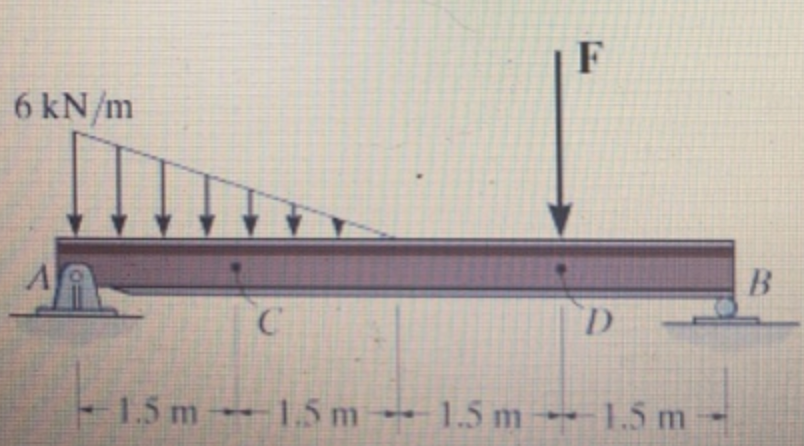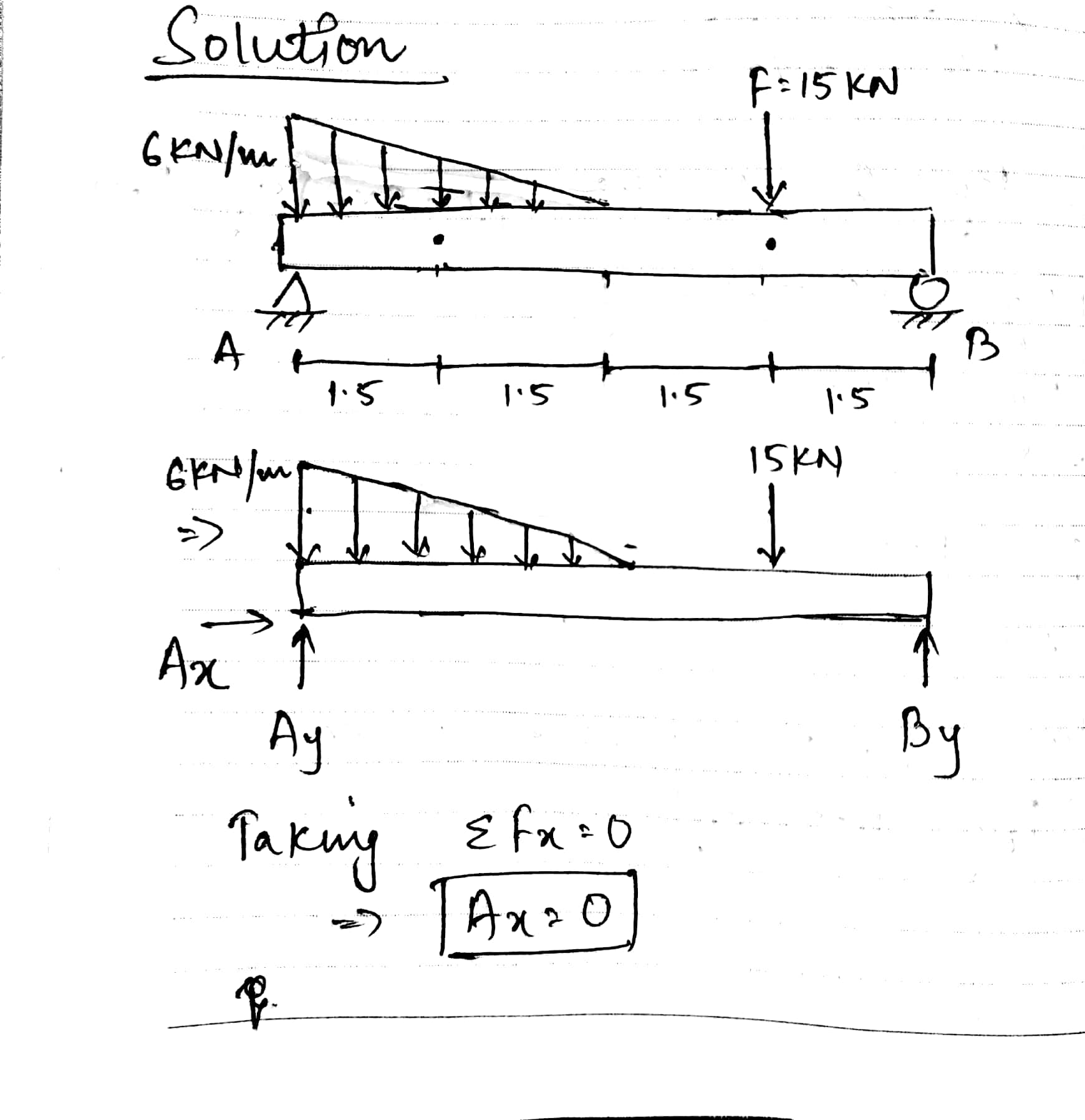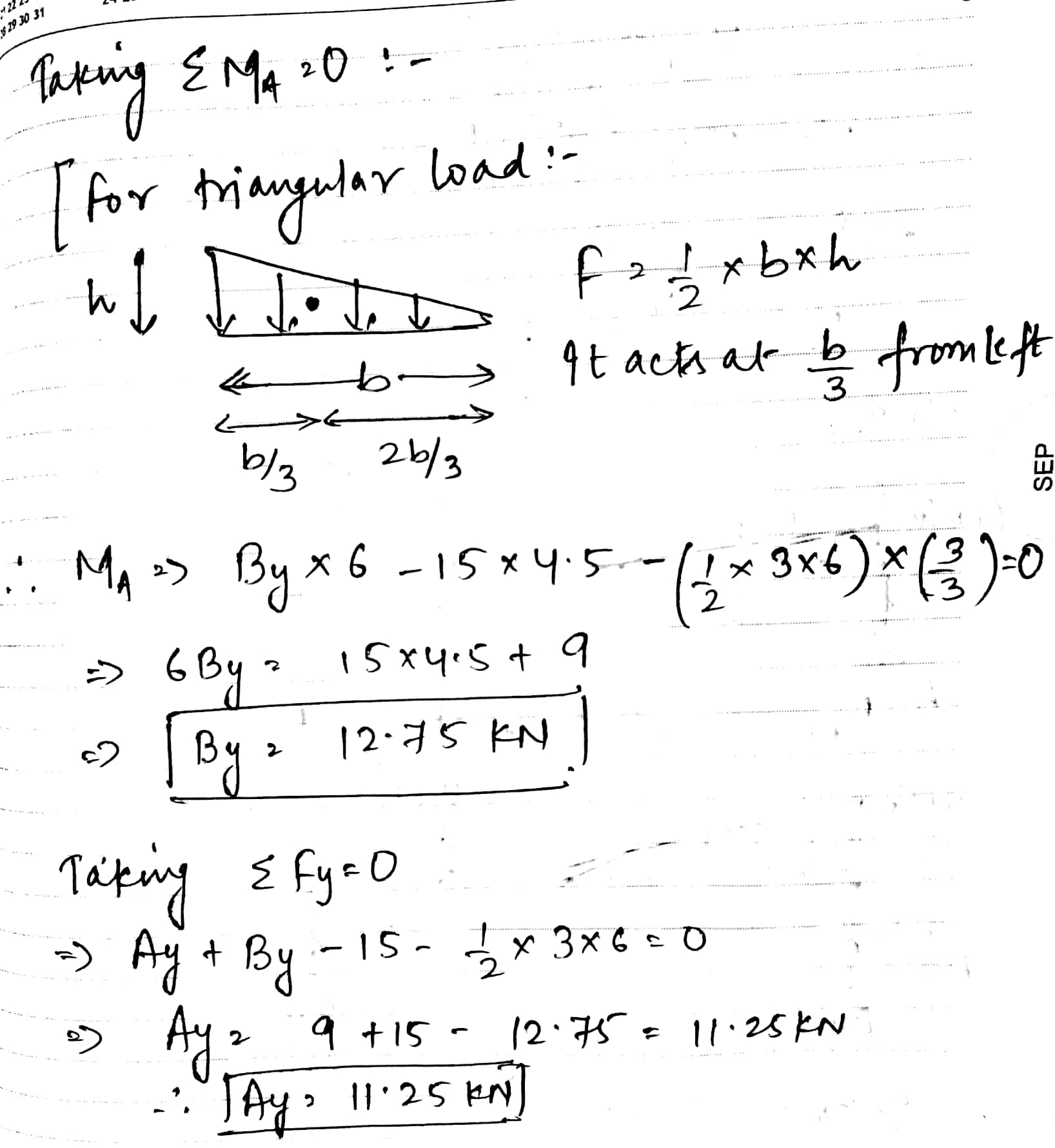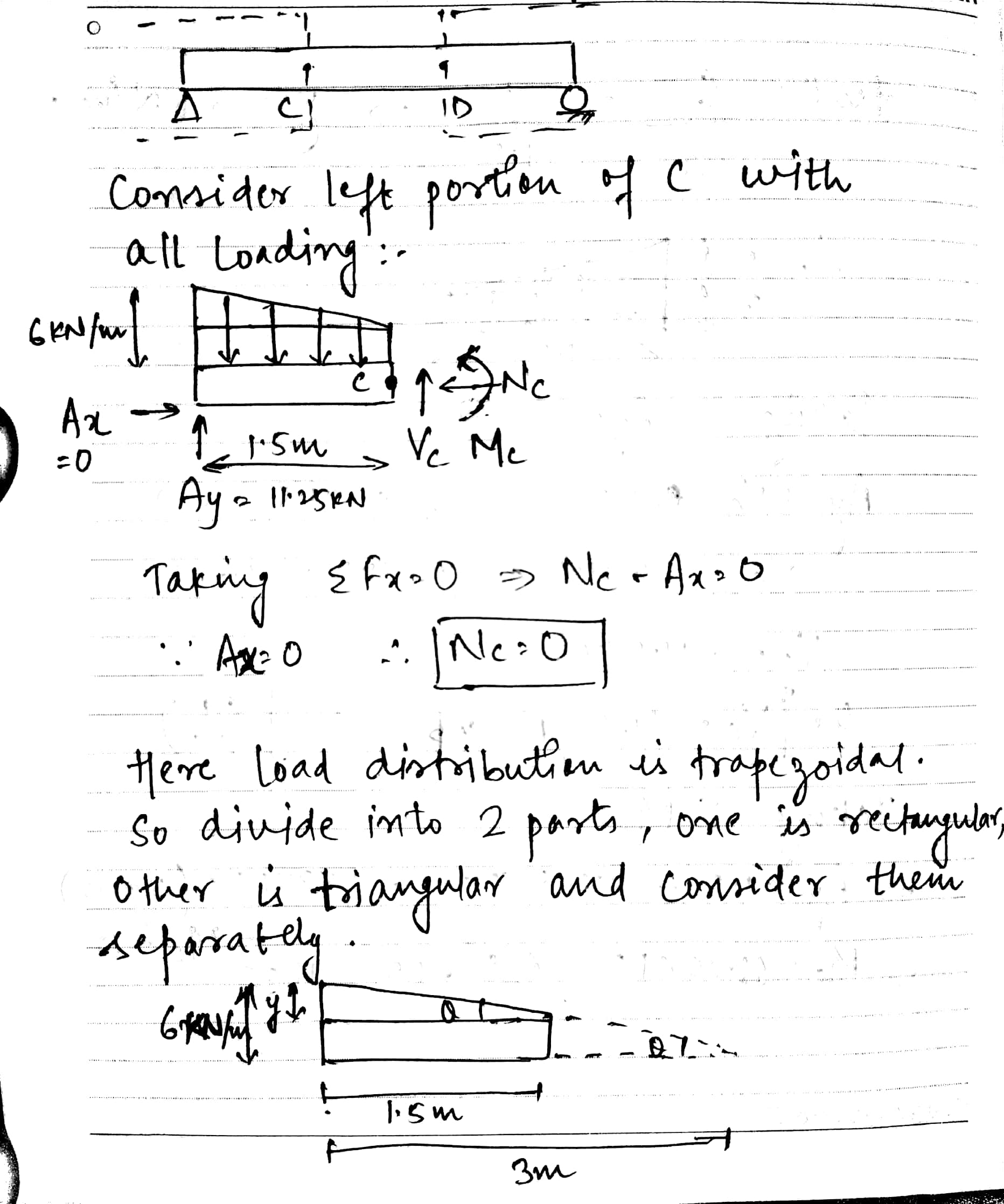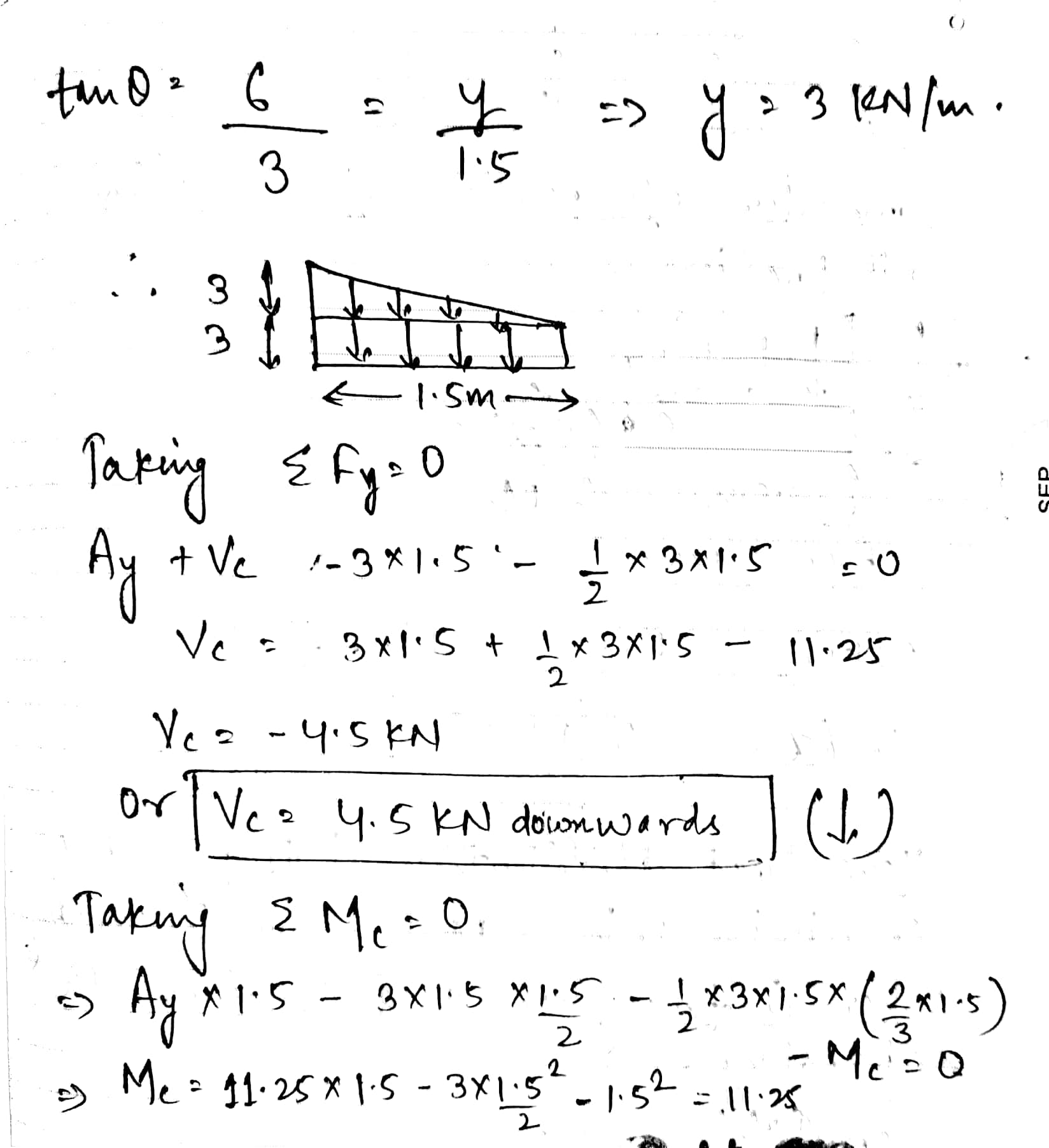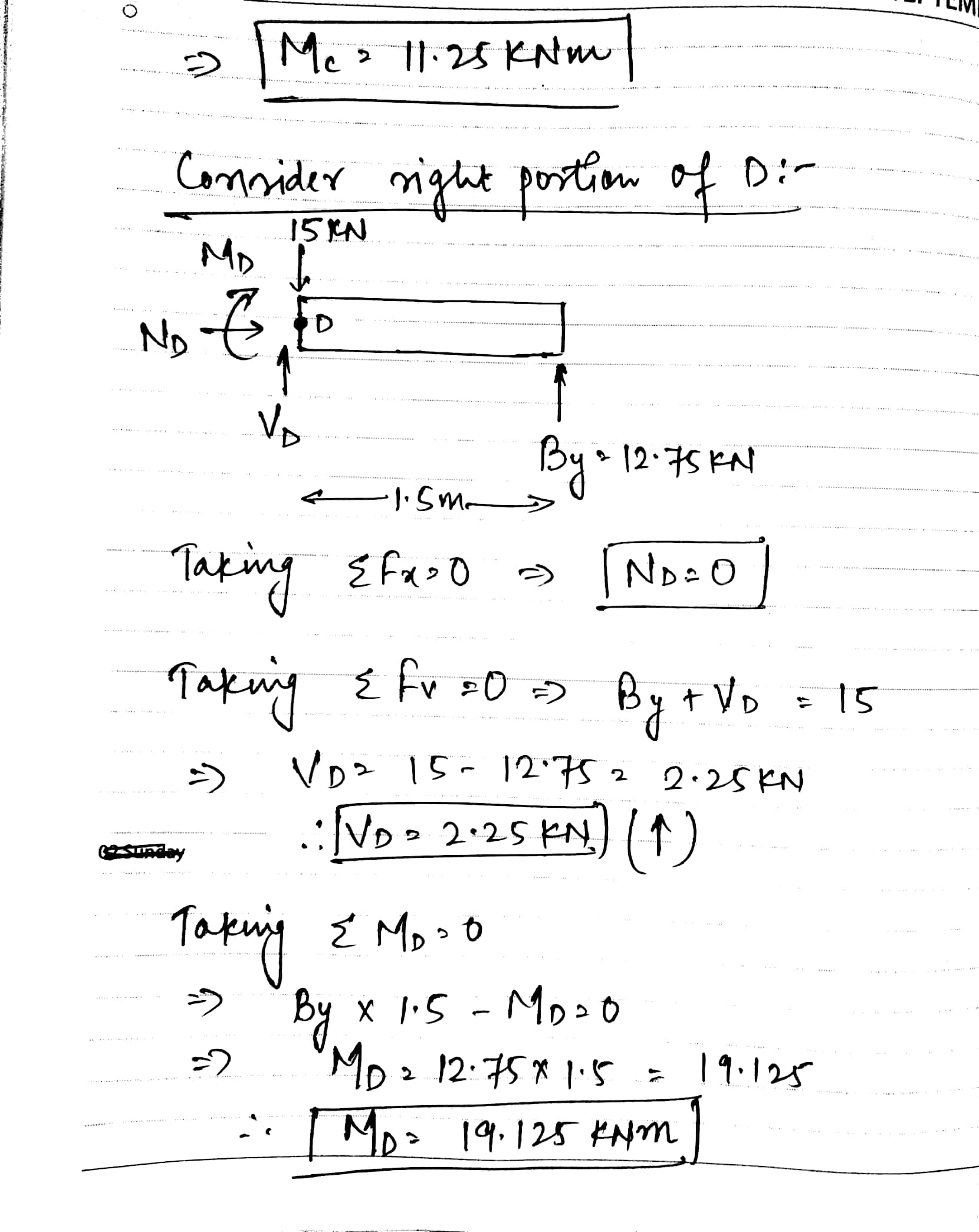#### Earn Coins

Coins can be redeemed for fabulous gifts.

Similar Homework Help Questions
• ### Determine the internal normal force, shear force, and moment at points and D in the simply supported beam.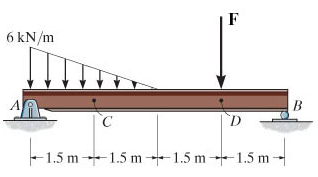Determine the internal normal force, shear force, and moment at points and D in the simply supported beam. Point Dis located just to the left of the F = 14 kN concentrated load.(Figure 1)

• ### Determine the internal normal force, shear force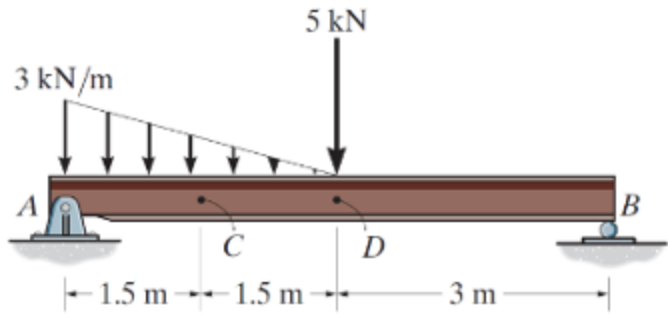Determine the internal normal force, shear force, and moment at points C and D in the simply supported beam using method of sections. Point D is A located just to the left of the 5-kN force. Also, draw shear and moment diagrams using the graphical method.

• ### Determine the internal force shear force and moment at point c. ENGR 2301 Statics Spring 2014 Name Exam 3 Sbow all w...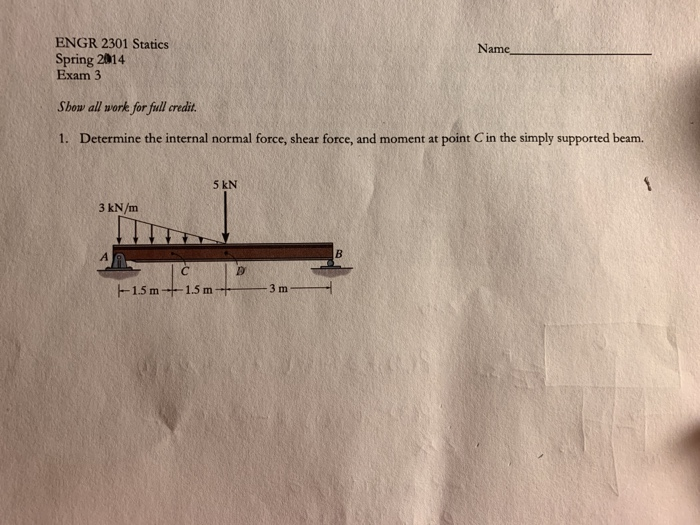Determine the internal force shear force and moment at point c. ENGR 2301 Statics Spring 2014 Name Exam 3 Sbow all work for full credit. 1. Determine the internal normal force, shear force, and moment at point C in the simply supported beam. 5 kN 3 kN/m --15 m -15 m3m

• ### 2) A beam supports the loading shown below. Determine the internal normal force, shear force and...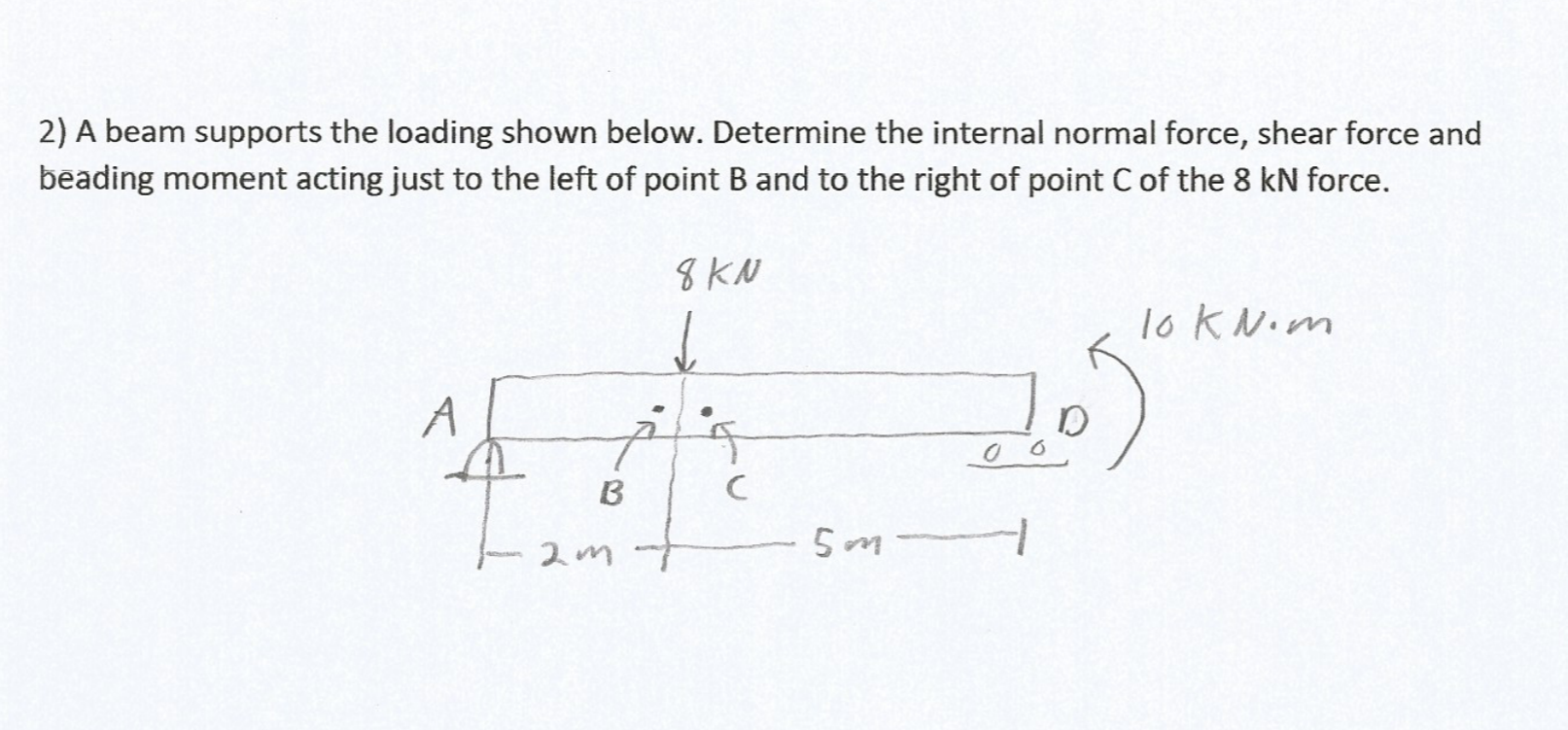2) A beam supports the loading shown below. Determine the internal normal force, shear force and beading moment acting just to the left of point B and to the right of point C of the 8 kN force. 8 KN loknom A D oo 6 ) B C 2m 5m 1

• ### Determine the internal normal force, shear force, and the moment at points C and D.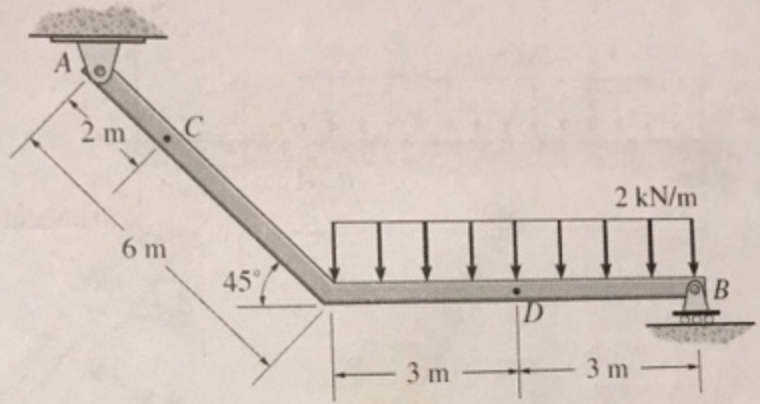Determine the internal normal force, shear force, and the moment at points C and D.

• ### 2) A beam supports the loading shown below. Determine the internal normal force, shear force and...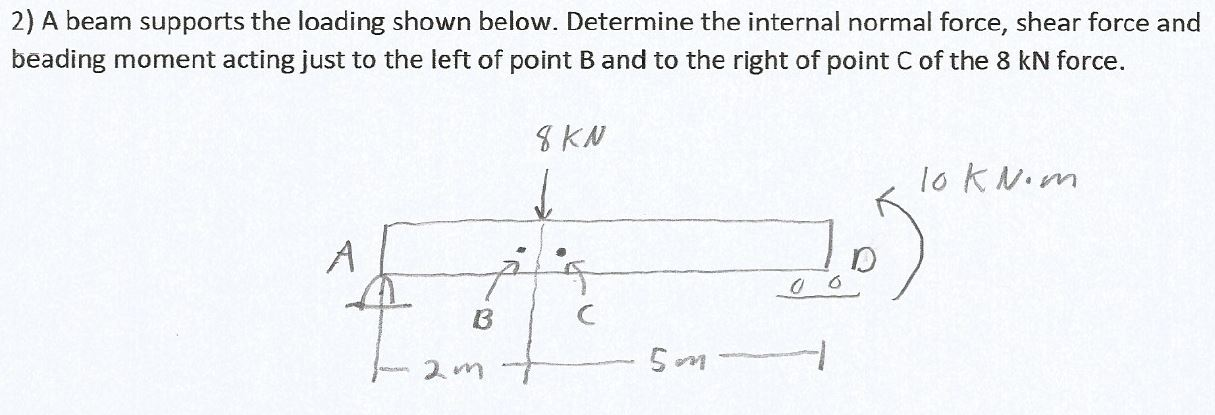2) A beam supports the loading shown below. Determine the internal normal force, shear force and beading moment acting just to the left of point B and to the right of point Cof the 8 kN force. 8 KN loknom A D t 0 0 B C 5m

• ### Determine the internal normal force, shear force, and bending moment at point C in the beam.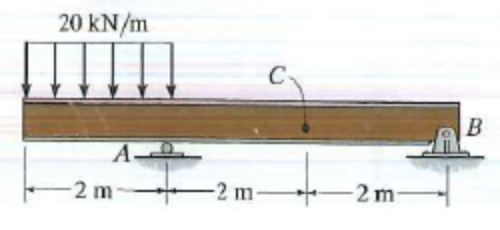Determine the internal normal force, shear force, and bending moment at point C in the beam.

• ### Determine the internal normal force, internal shear force, and internal moment at points D and E of the frame.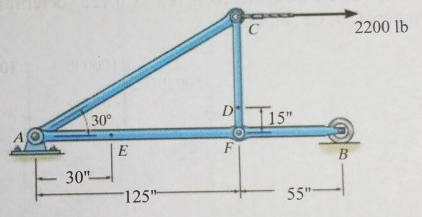Determine the internal normal force, internal shear force, and internal moment at points D and E of the frame.

• ### Determine the normal force, shear force, and moment in the beam at sections passing through points D and E. Point E is just to the right of the 3k load.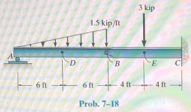Determine the normal force, shear force, and moment in the beam at sections passing through points D and E. Point E is just to the right of the 3k load.

• ### Question 2: A simply supported beam under loading as shown in Figure 1: 1. Draw the influence lines of the bending moment and shear force at point C (L/4) Using the influence lines to determine t...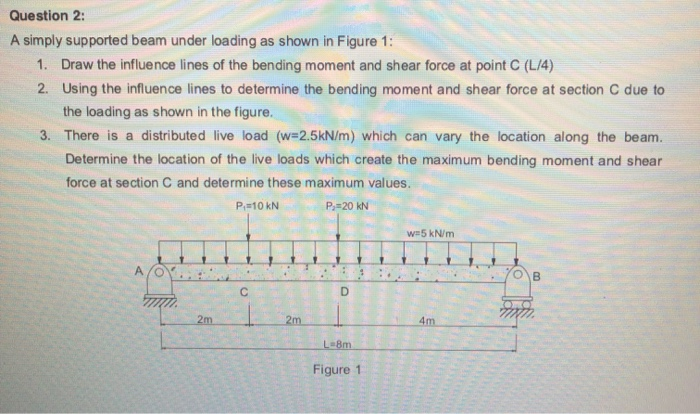Question 2: A simply supported beam under loading as shown in Figure 1: 1. Draw the influence lines of the bending moment and shear force at point C (L/4) Using the influence lines to determine the bending moment and shear force at section C due to the loading as shown in the figure. 2. 3. There is a distributed live load (w#2.5kN/m) which can vary the location along the beam. Determine the location of the live loads which create the...